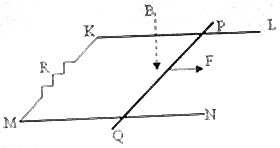## Pages

`“Life is like riding a bicycle.  To keep your balance you must keep moving.”–Albert Einstein`

## Saturday, December 15, 2007

### Answer to Free-Response Practice Question on Electromagnetic Induction

While answering free response questions never beat about the bush. Your effort should be to present things effectively in minimum words. At the same time, do not miss the required points. For instance, if you are asked to derive an expression, you should not write the final expression from your memory. You have to show the examiner, who evaluates your answers, that you know how to derive the expression, starting from basic things.
In the post dated 14th December 2007, the following free response question on electromagnetic induction was given to you for practice:The adjoining figure shows two smooth, straight, conducting, horizontal, parallel rails KL and MN on which a straight conducting rod PQ of mass ‘m’ can slide without friction, in a direction parallel to the rails. The rails and the rod have negligible resistance; but, there is a resistance R cunnected between the rails at one end. A uniform magnetic field of flux density B tesla is applied vertically downwards, throughout the region. The separation between the rails is L1 and the length of the rod PQ is L2.
(a) The rod PQ is pulled along the rails by a constant horizontal force F applied parallel to the rails. Derive an expression for the terminal velocity (vterminal) of the rod.
(b) If the rod starts from rest with an initial acceleration ‘a1’, find its acceleration when the velocity of the rod is vterminal/4.
(c) What is the power dissipated in the resistance R in terms of the magnetic field and other known parameters, when the terminal velocity is attained?
(d) If the rod PQ is stationary and the magnetic field B is decreasing, will the direction of the induced current in the loop KPQM be clockwise or anticlockwise, when viewed along the direction of the magnetic field? Give reasons for your answer.
As promised, I give below the answer:
(a) The motional emf induced in the portion of the rod between the rails = BL1v where ‘v’ is the velocity of the rod. (We have to consider the portion L1 since the induced current will flow in this portion only).
Magnetic force on the rod = IL1B where I is the induced current in the rod given by
I = BL1v/R.
When terminal velocity vterminal is attained, the applied force F is equal in magnitude (and opposite in direction) to magnetic force:
F = B2L12 vterminal/R, from which
vterminal = FR/ B2L12
(b) Initially since v =0, there is no magnetic force and therefore, F = ma1.
When the velocity is vterminal/4, the magnetic force = B2L12 vterminal/4R
The net force = F B2L12 vterminal/4R = ma.
Since F = B2L12 vterminal/R = ma1 , the above equation becomes
ma1 ma1/4 = ma from which a = 3a1/4.
(c) The power dissipated (P) when the rod moves with terminal velocity is
P = I2R = (BL1vterminal /R)2×R = B2L12 (vterminal) 2/R.
(d) By Lenz’s law, the induced current in the loop should oppose the change (decrement here) in the magnetic flux. Therefore, the induced current in this case has to produce a magnetic field inthe same direction as the external field B. This happens if the current flows in the clockwise direction.